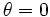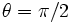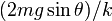# Block suspended from spring along a frictionless inclined plane

(diff) ← Older revision | Latest revision (diff) | Newer revision → (diff)
This article discusses a scenario/arrangement whose statics/dynamics/kinematics can be understood using the ideas of classical mechanics.
View other mechanics scenarios

The scenario here is a dry block (with a stable surface of contact) on a dry fixed frictionless inclined plane, with$\theta$ being the angle of inclination with the horizontal axis. In addition, there is a spring with one fixed end and the block attached at the other end, running parallel to the incline, so that if the block slides down the incline, the spring gets stretched and if the block slides up the incline, the spring gets compressed.

Denote by$k$ the spring constant of the spring -- we assume that the spring obeys Hooke's law.

The extremes for the angle$\theta$ are$\theta = 0$ (whence, the plane is horizontal), and$\theta = \pi/2$ (whence, the plane is vertical).

Though the picture depicts a square cross section for the block, this shape assumption is not necessary.

A more general scenario, that considers the possibility of friction along the inclined plane, is discussed at block suspended from spring along an inclined plane.

## Case that the spring is initially in the relaxed state

### Conclusion

In this case, the block and spring undergo simple harmonic motion. Note that the mean position is not the relaxed state of the spring, because of the role that gravitational force on the block plays in changing the equilibrium. Details about the oscillations are given below:

Quantity Value
Displacement of mean position of oscillations from usual relaxed state of spring$mg \sin \theta/k$
Amplitude of oscillation Also$(mg \sin \theta)/k$ (so the spring gets stretched by a maximum length of$(2mg \sin \theta)/k$ and thus oscillates between its relaxed position and a stretched position of$(2mg \sin \theta)/k$.
Angular frequency$\omega$ for oscillations$\sqrt{k/m}$
Frequency of oscillations (number of oscillations per second)$\frac{1}{2\pi} \sqrt{k/m}$
Time period of oscillation$2\pi \sqrt{m/k}$